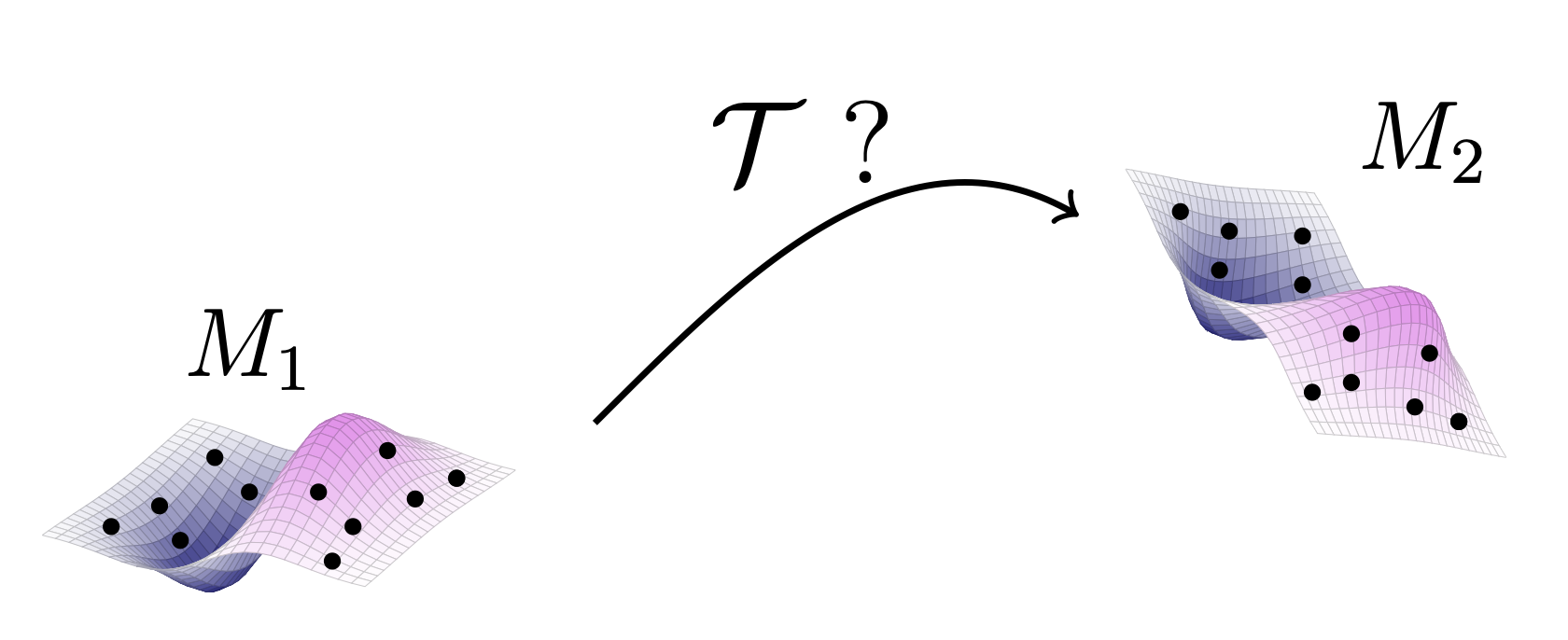# Research Projects

## Nonlinear matrix recovery

The problem of nonlinear matrix recovery consists in estimating a matrix based on incomplete observations (affine measurements), under the assumptions that the columns of the matrix satisfy a specific geometric structure. Structures where nonlinear matrix recovery is known to be efficient include data scattered in clusters and algebraic varieties (e.g. unions of several linear subspaces).

Our code is based on the resolution of a nonconvex optimization problem, using Riemannian optimization solvers. The code in both Matlab and Python is available on Github and is based on the research presented in this paper.

## Point cloud registration on algebraic varieties

The registration problem consists in finding a transformation between two point clouds which describe the same object in different coordinate systems.Our code is based on the resolution of a nonconvex optimization problem, using Riemannian optimization solvers. The code in both Matlab and Python is available on Github. This package is described in Chapter 5 of my doctoral thesis. A standalone paper is currently in preparation.